GFG App
Open AppBrowser
Continue

The List in CSS specifies the listing of the contents or items in a particular manner i.e., it can either be organized orderly or unorder way, which helps to make a clean webpage. It can be used to arrange the huge with a variety of content as they are flexible and easy to manage. The default style for the list is borderless. The list can be categorized into 2 types:

• Unordered List: In unordered lists, the list items are marked with bullets i.e. small black circles by default.
• Ordered List: In ordered lists, the list items are marked with numbers and an alphabet.

We have the following CSS lists properties, which can be used to control the CSS lists:

• list-style-type: This property is used to specify the appearance (such as disc, character, or custom counter style) of the list item marker.
• list-style-image: This property is used to set the images that will be used as the list item marker.
• list-style-position: It specifies the position of the marker box with respect to the principal block box.
• list-style: This property is used to set the list style.

### List Item Marker

This property specifies the type of item marker i.e. unordered list or ordered. The list-style-type property specifies the appearance of the list item marker (such as a disc, character, or custom counter style) of a list item element. Its default value is a disc.

Syntax:

`list-style-type:value;`

The following value can be used:

• circle
• decimal, eg :1,2,3, etc
• lower-roman
• upper-roman
• lower-alpha, eg: a,b,c, etc
• upper-alpha, eg: A, B, C, etc
• square

Example: This example describes the CSS List with the various list-style-type where the values are set to square & lower-alpha.

## HTML

 `` `<``html``>` `    ``<``head``>` `        ``<``style``>` `            ``ul.a {` `            ``list-style-type: square;` `            ``}` `            ``ol.c {` `            ``list-style-type: lower-alpha;` `            ``}` `        ``` `    ``` `    ``<``body``>` `        ``<``h2``>` `            ``GeeksforGeeks` `        ``` `        ``<``p``> Unordered lists ` `        ``<``ul` `class``=``"a"``>` `            ``<``li``>one` `            ``<``li``>two` `            ``<``li``>three` `        ``` `        ``<``ul` `class``=``"b"``>` `            ``<``li``>one` `            ``<``li``>two` `            ``<``li``>three` `        ``` `        ``<``p``> Ordered Lists ` `        ``<``ol` `class``=``"c"``>` `            ``<``li``>one` `            ``<``li``>two` `            ``<``li``>three` `        ``` `        ``<``ol` `class``=``"d"``>` `            ``<``li``>one` `            ``<``li``>two` `            ``<``li``>three` `        ``` `    ``` ``

Output: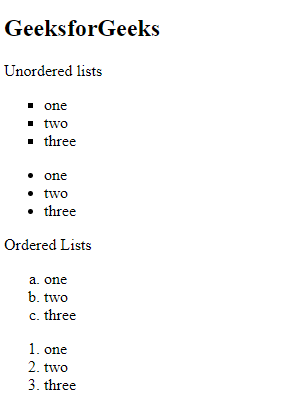### Image as List Marker

This property specifies the image as a list item marker. The list-style-image property is used to sets an image to be used as the list item marker. Its default value is “none”.

Syntax:

`list-style-image: url;`

Example: This example describes the CSS List with the various list-style-image where the values are set to url of the image.

## HTML

 `` `<``html``>` `    ``<``head``>` `        ``<``title``> CSS list-style-image Property ` `        ``<``style``>` `            ``ul {` `            ``list-style-image: url(` `            ``"https://contribute.geeksforgeeks.org/wp-content/uploads/listitem-1.png");` `            ``}` `        ``` `    ``` `    ``<``body``>` `        ``<``h1``>` `            ``GeeksforGeeks` `        ``` `        ``<``p``> Unordered lists ` `        ``<``ul``>` `            ``<``li``>1` `            ``<``li``>2` `            ``<``li``>3` `        ``` `    ``` ``

Output: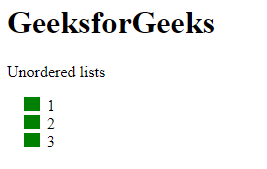### List Marker Position

This property specifies the position of the list item marker. The list-style-position property is used to sets the position of the marker relative to a list item. Its default value is “outside”.

There are 2 types of position markers:

• list-style-position: outside; In this, the bullet points will be outside the list item. The start of each line of the list will be aligned vertically.

Syntax:

`list-style-position: outside;`

Example: This example describes the CSS List with the various list-style-position where the value is set to outside.

## HTML

 `` `<``html``>` `    ``<``head``>` `        ``<``style``>` `            ``ul.a {` `            ``list-style-position: outside;` `            ``}` `        ``` `    ``` `    ``<``body``>` `        ``<``h2``>` `            ``GeeksforGeeks` `        ``` `        ``<``p``> Unordered lists ` `        ``<``ul` `class``=``"a"``>` `            ``<``li``>one` `                ``<``br``>` `                ``In this the bullet points will be outside the list item.` `            ``` `            ``<``li``>two` `                ``<``br``>` `                ``The start of each line of the list will be aligned vertically. ` `            ``` `            ``<``li``>three` `        ``` `    ``` ``

Output: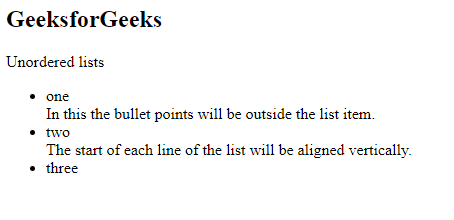• list-style-position: inside; In this, the bullet points will be inside the list. The line along with the bullet points will be aligned vertically.

Syntax:

`list-style-position: inside;`

Example: This example describes the CSS List with the various list-style-position where the value is set to inside.

## HTML

 `` `<``html``>` `    ``<``head``>` `        ``<``style``>` `            ``ul.a {` `            ``list-style-position: inside;` `            ``}` `        ``` `    ``` `    ``<``body``>` `        ``<``h2``>` `            ``GeeksforGeeks` `        ``` `        ``<``p``> Unordered lists ` `        ``<``ul` `class``=``"a"``>` `            ``<``li``>one` `                ``<``br``> ` `                ``In this the bullet points will be inside the list item.` `            ``` `            ``<``li``>two` `                ``<``br``> ` `                ``The line along with the bullet points will be aligned vertically.. ` `            ``` `            ``<``li``>three` `        ``` `    ``` ``

Output: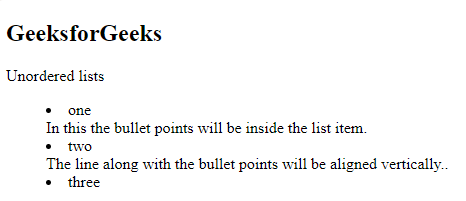### Shorthand Property

Shorthand Property allows us to set all the list properties in one command. The order of property is a type, position, and image. If any of the properties is missing, the default value is inserted.

Example: This example describes the CSS List using the shorthand property.

## HTML

 `` `<``html``>` `    ``<``head``>` `        ``<``style``>` `            ``ul.a {` `            ``list-style: square inside;` `            ``}` `        ``` `    ``` `    ``<``body``>` `        ``<``h2``>` `            ``GeeksforGeeks` `        ``` `        ``<``p``> Unordered lists ` `        ``<``ul` `class``=``"a"``>` `            ``<``li``>one` `            ``<``li``>two` `            ``<``li``>three` `        ``` `    ``` ``

Output: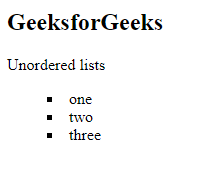Styling Lists: The list can be formatted in CSS. Different colors, borders, backgrounds, and paddings can be set for the lists.

Example: This example describes the CSS List where the various styling properties are applied to the element.

## HTML

 `` `<``html``>` `    ``<``head``>` `        ``<``style``>` `            ``ul.a {` `            ``list-style: square;` `            ``background: pink;` `            ``padding: 20px;` `            ``}` `        ``` `    ``` `    ``<``body``>` `        ``<``h2``>` `            ``GeeksforGeeks` `        ``` `        ``<``p``> Unordered lists ` `        ``<``ul` `class``=``"a"``>` `            ``<``li``>one` `            ``<``li``>two` `            ``<``li``>three` `        ``` `    ``` ``

Output: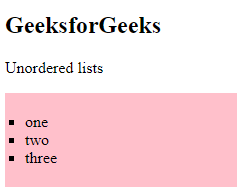Nested List: Lists can also be nested. We have sub-sections for sections, so we need the nesting of lists.

Example: This example describes the CSS List having a list declared inside another list.

## HTML

 `` `<``html``>` `    ``<``head``>` `    ``<``body``>` `        ``<``h2``>` `            ``GeeksforGeeks` `        ``` `        ``<``ul``>` `            ``<``li``>` `                ``one` `                ``<``ul``>` `                    ``<``li``>sub one` `                    ``<``li``>sub two` `                ``` `            ``` `            ``<``li``>` `                ``two` `                ``<``ul``>` `                    ``<``li``>sub one` `                    ``<``li``>sub two` `                ``` `            ``` `            ``<``li``>` `                ``three` `                ``<``ul``>` `                    ``<``li``>sub one` `                    ``<``li``>sub two` `                ``` `            ``` `        ``` `    ``` ``

Output: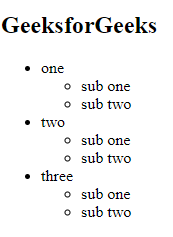Supported Browsers: